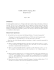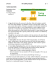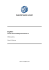# CMSC 28100-1 Spring 2015 Homework 2

## Transcription

CMSC 28100-1 Spring 2015 Homework 2
```CMSC 28100-1 Spring 2015
Homework 2
April 10, 2015
1. (HMU 8.2.2) Design Turing machines for the following languages:
(a) The set of strings with an equal number of 0’s and 1’s.
(b) {an bn cn | n > 1}
(c) {wwR | w is any string of 0’s and 1’s}, where wR is the reverse of a string. For instance,
10010R = 01001.
2. (HMU 8.2.3) Design a Turing machine that takes as input a number N in binary and adds
1 to it. To be precise, the tape initially contains a \$ followed by N in binary. The tape head is
initially scanning the \$ in state q0 . Your TM should halt with N +1, in binary, on its tape, scanning
the leftmost symbol of N + 1, in state qf . You may destroy the \$ in creating N + 1, if necessary.
For instance, q0 \$10011 7→ \$qf 10100 and q0 \$11111 7→ qf 100000.
(a) Give the transitions of your Turing machine, and explain the purpose of each state.
(b) Show the sequence of instantaneous descriptions (IDs) of your TM when given input \$111.
3. (HMU 8.2.5) Recall that the language L(M ) defined by a Turing machine M is the set of
input strings x such that M on input x halts in an accepting state. (M halts when there is no
entry in the transition function for the current state-symbol pair.)
Consider the Turing machine M with states {q0 , q1 , q2 , qf }, input alphabet {0, 1}, tape alphabet
{0, 1, B}, start state q0 , accepting state qf , and transition function δ. For each of the following
transition functions δ, informally but clearly describe the language L(M ) if δ consists of the following
set of rules.
(a)
δ(q0 , 0) = (q1 , 1, R)
δ(q1 , 1) = (q0 , 0, R)
δ(q1 , B) = (qf , B, R)
1
(b)
δ(q0 , 0) = (q0 , B, R)
δ(q0 , 1) = (q1 , B, R)
δ(q1 , 1) = (q1 , B, R)
δ(q1 , B) = (qf , B, R)
(c) (harder)
δ(q0 , 0) = (q1 , 1, R)
δ(q1 , 1) = (q2 , 0, L)
δ(q2 , 1) = (q0 , 1, R)
δ(q1 , B) = (qf , B, R)
4. (HMU 8.4.3) Informally but clearly describe nondeterministic Turing machines -multiple
tape if you like- that accept the following languages. Try to take advantage of nondeterminism
to avoid iteration and save time in the nondeterministic sense. That is, prefer to have your NTM
nondeterministically branch a lot, while each branch is short.
(a) The language of all strings of 0’s and 1’s that have some string of length 100 that repeats,
not necessarily consecutively. Formally, this language is the set of strings of 0’s and 1’s of the
form wxyxz where |x| = 100, and w, y, z are strings of arbitrary length (possibly zero).
(b) The language of all strings of the form w1 #w2 # · · · #wn for any n such that each wi is a
string of 0’s and 1’s, and for some j, wj is the integer j in binary.
(c) The language of all strings of the same form as (b), but for at least two values of j, we have
wj equal to j in binary.
2
```

### CMSC 28100-1 Spring 2015 Homework 3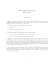### Basic Information - Tecnológico de Monterrey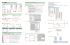### puzzle - Dallas Voice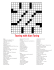### Installation instructions Finetune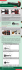### CSCE 4115/5400 EXAM #2 SAMPLE QUESTIONS SOLUTION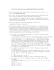### Sound Physics: The Piano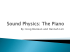### Online Official Mail-In Order Form Instructions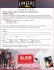### Min-ETune QUICK START GUIDE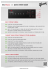### Homework 2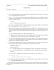### Enjoy The Journey Tangling Class with artist, Linda Swanekamp Art Gallery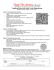### CMSC 28100-1 Spring 2015 Homework 5